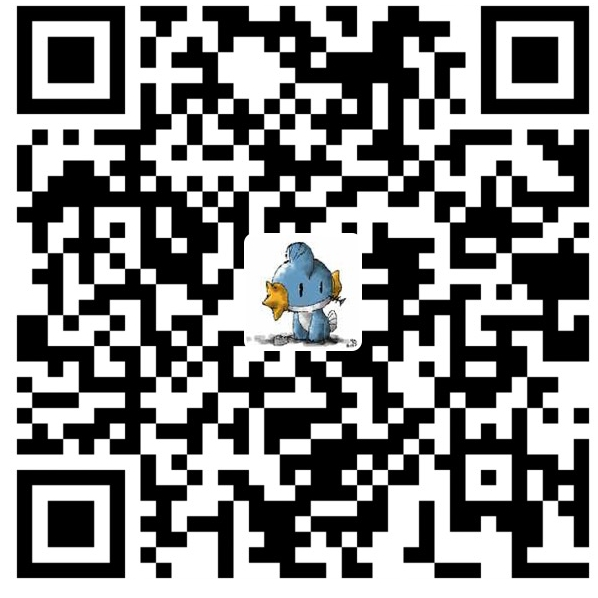# VBS基础教程第三篇

1)
Dim a,b,c
a=inputbox("a是:","输入半径")
b=Inputbox("b是:","输入半径")
c=a*2+b*2
Msgbox(c)

2)
Dim a,b,c
a=inputbox("a是:","输入半径")
b=Inputbox("b是:","输入半径")
c=(a+b)*2
Msgbox(c)

c=(int(a)+int(b))*2

dim a,b
a=true
b=false

dim a,b
a=12
b=13
if b>a then msgbox("B大于A")

dim a,b
a=12
b=13
if a<b then
msgbox("A小于B")
msgbox("B大于A")
end if

dim a
a=inputbox("请输入一个大于100的数")
a=int(a) 'inputbox返回的是字符串, 我们把他变成整数 : )
if a>100 then msgbox("正确")
if a<100 then msgbox("错误")

dim a
a=inputbox("请输入一个大于100的数")
a=int(a) 'inputbox返回的是字符串, 我们把他变成整数
if a>100 then
msgbox("正确")
else
msgbox("错误")
end if

dim a
a=inputbox("请输入一个大于100的数")
a=int(a) 'inputbox返回的是字符串, 我们把他变成整数
if a>100 then
msgbox("正确")
elseif a=100 then
msgbox("老大, 你耍我?")
else
msgbox("错误")
end if

Dim a,b,c,d
a=inputbox("a是:","输入半径")
b=Inputbox("b是:","输入半径")
d=Inputbox("答案:","输入答案")

c=a*2+b*2 '这里没有问题, 会自动转换
if d=c then
Msgbox("你好聪明")
else
Msgbox("你好猪头 自己的题还不会!")
end if

Dim a,b,c,d
a=inputbox("a是:","输入半径")
b=Inputbox("b是:","输入半径")
d=Inputbox("答案:","输入答案")
d=int(d)
'在这里我们取出了d的值, 变成整数, 在放回"d"这个盒子里
c=a*2+b*2
if d=c then
Msgbox("你好聪明")
else
Msgbox("你好猪头 自己的题还不会!")
end if

dim a,b
a=inputbox("输入一个数 >10")
b=inputbox("输入另一个数 >10")
a=int(a)
b=int(b)
if a>10 and b>10 then
msgbox("正确")
else
msgbox("错误")
end if

dim a,b
a=inputbox("输入一个数 >10")
b=inputbox("输入另一个数 >10")
a=int(a)
b=int(b)
if a>10 or b>10 then
msgbox("正确")
else
msgbox("错误")
end if

OK, 我们再来看一种新结构, 今天的课就结束, 已经午夜了, 我都累死了.

select case 变量名
case 值

case 值

case else

end select

dim a
a=inputbox("输入一个1--3的值")
a=int(a) '处理inputbox返回字符串的问题
select case a
case 1
msgbox("壹")
case 2
msgbox("贰")
case 3
msgbox("叁")
case else
msgbox("输入错误")
end select

dim a
a=inputbox("请输入1--3的值")
a=int(a)
if a=1 then
msgbox("壹")
elseif a=2 then
msgbox("贰")
elseif a=3 then
msgbox("叁")
else
msgbox("输入错误")
end if

OK, 今天到此结束, 总结一下:

1) inputbox返回的是一个字符串, 而不是一个数, 必须用a=int(a)这种形式转化成数

2) bool变量的值只有两种:true,false

2.5) and两边的表达式都是true, 则返回true. or两边的表达式有一个是true, 就返回true

3) if 语句的格式

4) select...case的格式

1) 使用3个bool值, 储存你的3兄弟姐妹是否是男性 (提示:sister1male=false)

2) 给定一个个数, 大于10而且小于20输出"正确", 否则输出"错误"

3) 输入12,或者15, 输出"正确", 否则输出"错误"

4) 把5以内的正整数都转换成中国大些数字

5) 自己随便设计一个程序, 应用今天的知识微信赞赏支付宝赞赏